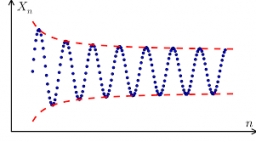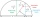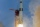# HP - harmonic progression 2

Compute the 16th term of the HP if the 6th and 11th term of the harmonic progression are 10 and 18 respectively.

h16 =  90

### Step-by-step explanation:Did you find an error or inaccuracy? Feel free to write us. Thank you!Tips to related online calculators
Looking for calculator of harmonic mean?
Looking for a statistical calculator?

## Related math problems and questions:

• Arithmetic progression 2The 3rd term of an Arithmetic progression is ten more than the first term, while the fifth term is 15 more than the second term. Find the sum of the 8th and 15th terms of the Arithmetic progression if the 7th term is seven times the first term.
• HP - harmonic progressionDetermine the 10th term of the harmonic progression 6,4,3,…
• Insert 7Insert five harmonic means between 3 and 18
• PoolIf water flows into the pool by two inlets, fill the whole for 19 hours. The first inlet filled pool 5 hour longer than the second. How long pool take to fill with two inlets separately?
• HP - harmonic progressionDetermine the 8th term of the harmonic progression 2, 4/3, 1,…
• Harmonic meanIf x, y, z form a harmonic progression, then y is the harmonic mean of x and z. Find the harmonic mean of the numbers 6 and 5.
• Harmonic seriesInsert four members between 5/3 and 5/11 to form harmonic series (means).
• What is 10What is the 5th term, if the 8th term is 80 and common ratio r =1/2?
• Find the 19Find the 1st term of the GP ___, -6, 18, -54.
• Speed of Slovakian trainsRudolf decided to take the train from the station 'Ostratice' to 'Horné Ozorovce'. In the train timetables found train Os 5409 : km 0 Chynorany 15:17 5 Ostratice 15:23 15:23 8 Rybany 15:27 15:27 10 Dolné Naštice 15:31 15:31 14 Bánovce nad Bebravou 15:35 1
• Find d 2Find d in an A. P. whose 5th term is 18 and 39th term is 120.
• If the 3If the 6th term of a GP is 4 and the 10th is 4/81, find common ratio r.
• Sequence 11What is the nth term of this sequence 1, 1/2, 1/3, 1/4, 1/5?
• GP membersThe geometric sequence has 10 members. The last two members are 2 and -1. Which member is -1/16?
• 15th term of APWhat is the 15th term; x1=1.5, d=4.5?
• 10th termWhat is the 10th term of the Arithmetic Progression if x1=4 and d=5?
• Common differenceThe 4th term of an arithmetic progression is 6. If the sum of the 8th and 9th terms is -72, find the common difference.